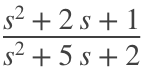## Nodal Problem

% Define symbols
syms s R1 R2 L C V Vc Vx
% Enter each equation on a line
eqn1 = (Vx-V)/R1 + Vx/(L*s) + (Vx-Vc)/R2 == 0;
eqn2 = (Vc-Vx)/R2 + Vc/(1/(C*s)) == 0;
% Use Matlab to solve the equations for Vx and Vc
[solVx,solVc]=solve([eqn1,eqn2],[Vx,Vc])
solVx =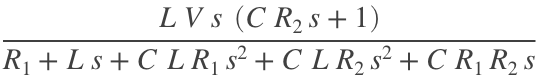solVc =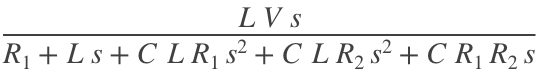% The transfer func G = I2(s)/V(s) is
G = solVc/V;
% To make it pretty, we can use collect() to group the coefficients of the
% polynomial before printing
collect(G,s)
ans =## Inverting Op Amp

% Define symbols
syms s R1 R2 C2 C1
% Define feedback impedance
Zf=R2+1/(C2*s)
Zf =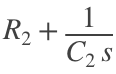% Define input impedance
Zi=1/(1/R1+C1*s)
Zi =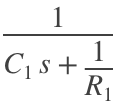% Get transfer function, G
G=-Zf/Zi
G =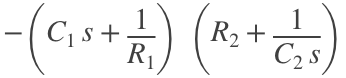% Collect terms of transfer function to simplify
G=collect(G)
G =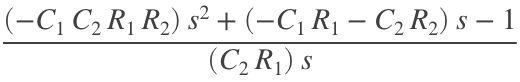% substitute numbers
G = subs(G,[C1,C2,R1,R2],[5.6e-6,0.1e-6,360e3,220e3]);
% display using decimals instead of fractions
vpa(G,5)
ans =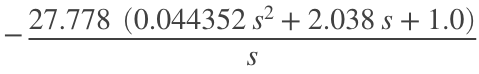## Non-Inverting Op Amp

% Define symbols
syms s R1 R2 C2 C1
% Define feedback impedance
Zf=1/(1/R2+C2*s)
Zf =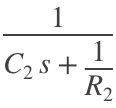% Define input impedance
Zi=R1+1/(C1*s)
Zi =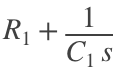% Get transfer function, G
G=1+Zf/Zi
G =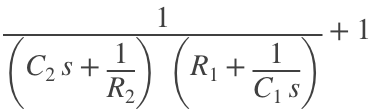% Collect terms of transfer function to simplify
G=collect(G)
G =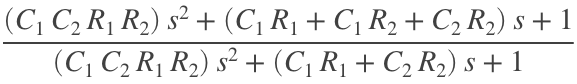## Exercise 2.6 (nodal)

% Define symbols
syms s V Vl Vx
% Enter each equation on a line
eqn1 = (Vx-V)/1 + Vx/s + (Vx-Vl)/1 == 0;
eqn2 = (Vl-V)/s + (Vl-Vx)/1 + Vl/s == 0;
% Use Matlab to solve the equations for Vx and Vc
[solVx,solVl]=solve([eqn1,eqn2],[Vx,Vl])
solVx =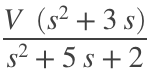solVl =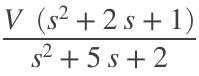G=solVl/V
G =